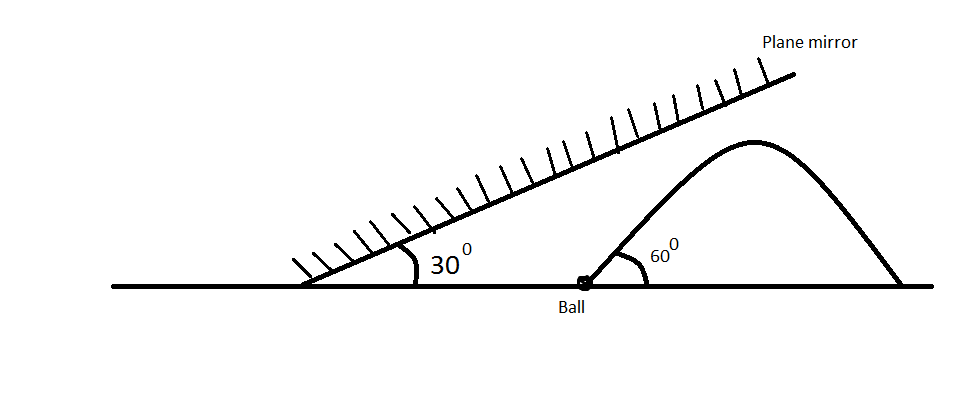Optics + Projectile motion + Relative motionA ball is thrown with speed 20 m/s at an angle of 60 degree with the horizontal and a big plane mirror is inclined at an angle 30 degree with the horizontal as shown in the figure. Find the time at which the image of ball appears to be at rest relative to the object. The answer is of the form (a/root b) then the value of a+b is:

×